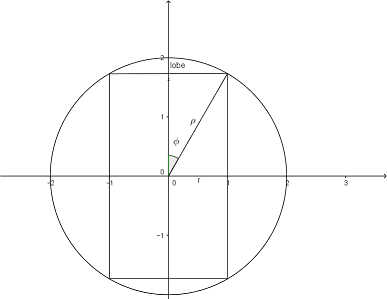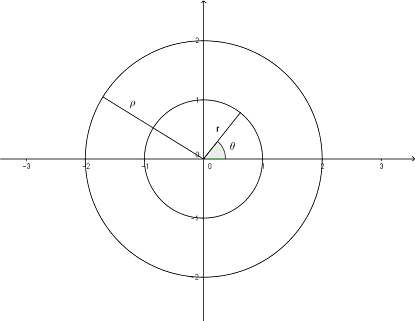# A sphere with a hole through it (a triple integral).

• TheSodesa

## Homework Statement

A sphere has a diameter of ##D = 2\rho = 4cm##. A cylindrical hole with a diameter of ##d = 2R = 2 cm## is bored through the center of the sphere. Calculate the volume of the remaining solid. (Spherical or cylindrical coordinates?)

hint: Place the shape into a convenient place in the xyz-coordinate system.

## Homework Equations

Cylindrical coordinates:
\begin{cases}
x = rcos(\theta)\\
y = rsin(\theta)\\
z = z\\
x^2+y^2 = r^2\\
dV_c = rdzdrd\theta
\end{cases}
Spherical coordinates:
\begin{cases}
x = rcos(\theta) = \rho sin(\phi)cos(\theta)\\
y = rsin(\theta) = \rho sin(\phi)sin(\theta)\\
z = \rho cos(\phi)\\
x^2 + y^2 + z^2 = \rho^2\\
dV_s = \rho^2 sin(\phi)d\rho d\phi d\theta
\end{cases}

## The Attempt at a Solution

I looked around the internet and found the exact same problem, except in that case the presenter of the question was kind enough to point out that this would be easier to do in cylindrical coordinates. In any case, my guess is that I should try to calculate the volume of the hole and subtract it from the volume of the sphere which we know to be ##V_s = \frac{4}{3}\pi \rho^3##. The hole seems to consist of a cylinder, with two lobes attached at each end.

Let us place the sphere's center at the origin (note that the small r in the pictures should be ##R## considering our derivation of the volume below):If we first try cylindrical coordinates, we can easily calculate the volume of the cylinder:

\begin{align*}
V_c
&= \int_{0}^{2\pi}\int_{0}^{\pi}\int_{-\sqrt{\rho^2 - R^2}}^{\sqrt{\rho^2 - R^2}}rdzdrd\theta\\
&= \int_{0}^{2\pi}\int_{0}^{\pi} 2R\sqrt{\rho^2 - R^2}drd\theta\\
&= \int_{0}^{2\pi} R^2\sqrt{\rho^2 - R^2}d\theta\\
&= 2 \pi R^2\sqrt{\rho^2 - R^2}
\end{align*}
As for the lobes, they are limited by
\begin{cases}
0 \leq \theta \leq 2\pi\\
0 \leq r \leq R\\
\sqrt{\rho^2 - R^2} \leq z \leq \sqrt{\rho^2 - r^2}
\end{cases}
Then the volume of our lobe is
\begin{align*}
V_L
&= \int_{0}^{2\pi}\int_{0}^{R}\int_{\sqrt{\rho^2 - R^2}}^{\sqrt{\rho^2 - r^2}} r dz dr d\theta \\
&= \int_{0}^{2\pi}\int_{0}^{R} r \left( \sqrt{\rho^2 - r^2}-\sqrt{\rho^2 - R^2} \right) dr d\theta\\
&= \int_{0}^{2\pi}\left(\int_{0}^{R} r\sqrt{\rho^2 - r^2} dr - \int_{0}^{R} r (\sqrt{\rho^2 - R^2} dr\right) d\theta\\
&= \int_{0}^{2\pi}
\left(\int_{0}^{R}\frac{-1}{2}\times \frac{2}{3}\times \frac{3}{2}\times -2r\sqrt{\rho^2 - r^2} dr
- \int_{0}^{R} r \stackrel{constant}{\sqrt{\rho^2 - R^2}} dr\right)
d\theta\\
&= \int_{0}^{2\pi} \left[ \frac{-2}{6}\sqrt{\rho^2 - r^2}^3\right]_0^R - \frac{1}{2}R^2\sqrt{\rho^2 - R^2} d\theta\\
&= \int_{0}^{2\pi} \frac{-1}{3}\sqrt{\rho^2 - r^2}^3 + \frac{1}{3}\rho^3 - \frac{1}{2}R^2\sqrt{\rho^2 - R^2}\ d\theta\\
&= \frac{-2\pi}{3}\sqrt{\rho^2 - r^2}^3 + \frac{2\pi}{3}\rho^3 - \frac{2\pi}{2}R^2\sqrt{\rho^2 - R^2}
\end{align*}
Now since in the case of our problem ##\rho = 2R##,
\begin{equation*}
\sqrt{\rho^2 - R^2} = \sqrt{(2R)^2 - R^2} = \sqrt{3R^2} = \sqrt{3}R
\end{equation*}
so
\begin{align*}
V_L
&= \frac{-2\pi}{3}\sqrt{\rho^2 - r^2}^3 + \frac{2\pi}{3}\rho^3 - \frac{2\pi}{2}R^2\sqrt{\rho^2 - R^2}\\
&= \frac{-2\pi}{3}(\sqrt{3}R)^3 + \frac{2\pi}{3}(2R)^3 - \pi R^2(\sqrt{3}R)\\
&= \pi R^3 \left( \frac{-6\sqrt{3}}{3} + \frac{16}{3} - \frac{3\sqrt{3}}{3}\right)\\
&=\frac{\pi R^3}{3}(16 - 9\sqrt{3})
\end{align*}
Then our volumes are:
\begin{cases}
Sphere: V_s &= \frac{4}{3}\pi \rho^3 \stackrel{\rho = 2R}{=} \frac{32}{3}\pi R^3\\
Cylinder: V_c &= 2\pi R^2 \sqrt{\rho^2 - R^2} = 2\sqrt{3}\pi R^3\\
Two \ lobes: 2V_L &= \frac{2\pi R^3}{3}(16 - 9\sqrt{3})
\end{cases}
and our total volume
\begin{align*}
V
&= V_s - V_c - 2V_L\\
&= \frac{32}{3}\pi R^3 - 2\sqrt{3}\pi R^3 - \frac{2\pi R^3}{3}(16 - 9\sqrt{3})\\
&= \frac{\pi R^3}{3} \left(32 - 2\sqrt{3} - 32 + 9\sqrt{3} \right)\\
&= \frac{12\sqrt{3}\pi R^3}{3}\\
&= 4\pi \sqrt{3} R^3\\
&\stackrel{R=1}{=} 4\pi \sqrt{3} \ (cm^3)
\end{align*}
which is the correct answer according to the question sheet.

Boom. Shaka. Laka.

I might get back to solving the spherical case in the future, but no way am I doing that now. This took me long enough with all the mistakes I made along the way and I have two more problems I need to get done by Monday.

Last edited:

Did you have a question?

Did you have a question?
I did. I just managed to figure it out it partway through typing this, so I figured I'd post it anyway. I just marked this as solved.

The limits on your integrals for calculating the volume of the cylinder are messed up. Perhaps just a typo, though I'm not sure where that 2R came from. And why did you set R=1 at the end when R=2 cm?

In any case, wouldn't it be more straightforward to not break it into pieces and just calculate the volume of the hole in one shot, perhaps using the method of cylinders?

The limits on your integrals for calculating the volume of the cylinder are messed up. Perhaps just a typo, though I'm not sure where that 2R came from. And why did you set R=1 at the end when R=2 cm?

In any case, wouldn't it be more straightforward to not break it into pieces and just calculate the volume of the hole in one shot, perhaps using the method of cylinders?
Whoops. I changed my notation partway through the problem and forgot to change the original symbols to match.
Ninja editing...P.S. I was specifically supposed to use triple integrals to solve this.

It is a remarkable fact that if a cylindrical hole is bored through the centre of a sphere, and the length of the hole is 2d (as measured along the remaining material) then the volume of material remaining in the sphere is ##\frac 43\pi d^3##. This can be seen by taking a slice perpendicular to the hole's axis. It is not hard to show that the area of the annulus depends only on d and the distance from the sphere's centre to the slice.
No integrals necessary, beyond the standard result for the volume of a sphere.